CONTINUITY OF FUNCTIONS OF ONE VARIABLE

The following problems involve the CONTINUITY OF A FUNCTION OF ONE VARIABLE. Function y = f(x) is continuous at point x=a if the following three conditions are satisfied :

i.) f(a) is defined ,

ii.)exists (i.e., is finite) ,

and

iii.).

Function f is said to be continuous on an interval I if f is continuous at each point x in I. Here is a list of some well-known facts related to continuity :

1. The SUM of continuous functions is continuous.

2. The DIFFERENCE of continuous functions is continuous.

3. The PRODUCT of continuous functions is continuous.

4. The QUOTIENT of continuous functions is continuous at all points x where the DENOMINATOR IS NOT ZERO.

5. The FUNCTIONAL COMPOSITION of continuous functions is continuous at all points x where the composition is properly defined.

6. Any polynomial is continuous for all values of x.

7. Function ex and trigonometry functionsandare continuous for all values of x.

Most problems that follow are average. A few are somewhat challenging. All limits are determined WITHOUT the use of L'Hopital's Rule. If you are going to try these problems before looking at the solutions, you can avoid common mistakes by using the above step-by-step definition of continuity at a point and the well-known facts, and by giving careful consideration to the indeterminate formduring the computation of limits. Knowledge of one-sided limits will be required. For a review of limits and indeterminate forms click here.

• PROBLEM 1 : Determine if the following function is continuous at x=1 .• PROBLEM 2 : Determine if the following function is continuous at x=-2 .• PROBLEM 3 : Determine if the following function is continuous at x=0 .• PROBLEM 4 : Determine if the function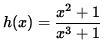is continuous at x=-1 .

• PROBLEM 5 : Check the following function for continuity at x=3 and x=-3 .• PROBLEM 6 : For what values of x is the functioncontinuous ?

• PROBLEM 7 : For what values of x is the function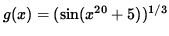continuous ?

• PROBLEM 8 : For what values of x is the function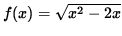continuous ?

• PROBLEM 9 : For what values of x is the function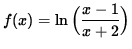continuous ?

• PROBLEM 10 : For what values of x is the function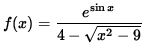continuous ?

• PROBLEM 11 : For what values of x is the following function continuous ?• PROBLEM 12 : Determine all values of the constant A so that the following function is continuous for all values of x .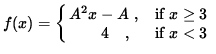• PROBLEM 13 : Determine all values of the constants A and B so that the following function is continuous for all values of x .• PROBLEM 14 : Show that the following function is continuous for all values of x .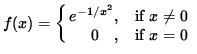• PROBLEM 15 : LetShow that f is continuous for all values of x . Show that f is differentiable for all values of x, but that the derivative, f' , is NOT CONTINUOUS at x=0 .